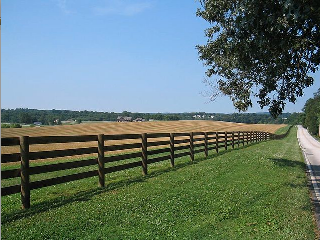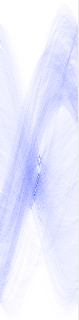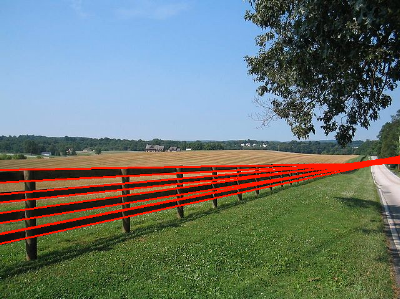## Friday, May 3, 2013

### Hough Transformation - C++ Implementation

The Hough Transformation is a great way to detect lines in an image and it is quite useful for a number of Computer Vision tasks.

A good definition can be found at http://en.wikipedia.org/wiki/Hough_transform

This post will guide you through (a little) theory and focusses on the C++ implementation of the linear Hough Transformation.

### Theory

Let's take an image (Fig 1) with two lines A and B. Obviously both lines are each made of its own set of pixels laying on a straight line. Now, one way or another we need to learn our software which pixels are on a straight line and, if so, to what line they belong to.

The trick of the Hough Transformation is to represent lines in a polar coordinate system (see Fig 2), or in this case, also called "Hough Space".

You can read this wiki for more details but important in Fig 2 is that we imagine a line from the center (the blue line) which is perpendicular to the line we want to find (the green line) and we call the distance from the center r and θ(theta) is the angle to the X axis (the angle between the blue line and the X axis).

The transformation from any (x, y) pixel in Fig1 to the (r, θ) representation int Fig2 is the following:

r = x.cos(θ) + y.sin(θ)

Now, if you lean back and think a bit about Fig2. Every possible line can be represented by a unique r and θ. And further, every pixel on a given line will transform to the exact same r and θ for that line. And this is immediately the most important aspect of the whole Hough stuff. If you get that, you understand the transformation.

So, given the above; every line in polar space is defined by its unique coordinates (r, θ). Let play with this and say we decide to use  θ ∈ { 1 ... 180 } with a resolution of 1 degree. 1 to 180 allow us to make any line if we allow r to be negative. Check Fig2, if we use the same θ but make r negative that line A' would be at the oposite side (left) and parallel to A.

That means that for every point (x,y) we can compute 180 r values.
 (x1,y1) (x2,y2) r1_1 = x1.cos(1) + y1.sin(1) r1_2 = x1.cos(2) + y1.sin(2) r1_3 = x1.cos(3) + y1.sin(3) ... r1_180 = x1.cos(180) + y1.sin(180) r2_1 = x2.cos(1) + y2.sin(1) r2_2 = x2.cos(2) + y2.sin(2) r2_3 = x2.cos(3) + y2.sin(3) ... r2_180 = x2.cos(180) + y2.sin(180)

So far, all fine, but to make it really useful we need to add one extra element and that is called an Accumulator. An Accumulator is nothing more than a 2 dimensional matrix with θmax columns and rmax rows. So each cell, or bin, represents a unique coordinate (r, θ) and thus one unique line in Hough Space.

The algorithm is as follow:

• For every point in your image
• compute r for θ = 1 to 180
• increment the Accumulator by 1 for the every found r, so increment bin(r, θ)Fig 3 - Illustration

Once done, the accumulator bins contain a count of pixels for every possible line. Go over the binss and only keep the ones that are over a certain threshold (for example, you can decide to only consider it a real line if it contains at least 80 pixels).

The result is a set of coordinates (r, θ), one for every straight line in the image thats above the threshold. And these polar coordinates easily can be computed back to 2 points in the original image space, just solve the equation for x and y.### C++ Implementation

The following code is a command line implementation that take an image path as input (png, jpg, ...) and displays a view with the detected lines drawn over the image. It also show a view of the accumulator, which can look pretty neat.

The procedure is as follow
2. Do Canny edge detection on it  so we have a BW image with lines and points representing the edges
3. Do a hough transformation on the edge image
4. Search the accumulator for all lines given a certain threshold
5. Visualize all on the screen
Since we focus on the implementation of step 3 & 4 (Hough) we are going to use some open source for step 1, 2 and 5. I decided to use OpenCV (http://opencv.org), its open source, popular, awesome and pretty easy to use.

OpenCV itself has a Hough Transformation, but we are not going to use that, our goal is to get insight in the inner working of the transformation and implement it ourselves. Also I think OpenCV implemented a Probabilistic or Randomized Hough Transform, wich is a faster but different approach http://en.wikipedia.org/wiki/Randomized_Hough_transform'.

#### 1, 2 - Load the image and do the edge detection

Let's work with the following image:Original Image

``````1:       cv::Mat img_edge;
2:       cv::Mat img_blur;
3:
4:       cv::Mat img_ori = cv::imread( file_path, 1 );
5:       cv::blur( img_ori, img_blur, cv::Size(5,5) );
6:       cv::Canny(img_blur, img_edge, 100, 150, 3);
7:
8:       int w = img_edge.cols;
9:       int h = img_edge.rows;
``````

This is all OpenCV stuff, line 4 loads the image from path 'file_path'. Line 5 blurs it a bit and line 6 does the actual Edge detection. The result is a Black and White image 'img_edge' with the edges.Canny Edge Detection

#### 3 - The Hough Transformation

The following code does the actual transformation, img_data contains the edge data. The function loops over all pixels in the edge image and increments the accumulator at  the computed (r, θ).

``````1:       int Hough::Transform(unsigned char* img_data, int w, int h)
2:       {
3:            _img_w = w;
4:            _img_h = h;
5:
6:            //Create the accu
7:            double hough_h = ((sqrt(2.0) * (double)(h>w?h:w)) / 2.0);
8:            _accu_h = hough_h * 2.0; // -r -> +r
9:            _accu_w = 180;
10:
11:            _accu = (unsigned int*)calloc(_accu_h * _accu_w, sizeof(unsigned int));
12:
13:            double center_x = w/2;
14:            double center_y = h/2;
15:
16:
17:            for(int y=0;y<h;y++)
18:            {
19:                 for(int x=0;x<w;x++)
20:                 {
21:                      if( img_data[ (y*w) + x] > 250 )
22:                      {
23:                           for(int t=0;t<180;t++)
24:                           {
25:                                double r = ( ((double)x - center_x) * cos((double)t * DEG2RAD)) + (((double)y - center_y) * sin((double)t * DEG2RAD));
26:                                _accu[ (int)((round(r + hough_h) * 180.0)) + t]++;
27:                           }
28:                      }
29:                 }
30:            }
31:
32:            return 0;
33:       }
34:
``````

Line 11 creates the empty accumulator, we have chosen to compute r for θ = {1..180} so the width contain 180 bins, the maximum height depends on the image size and is computed at line 7.

Line 17 to 30 loops over every point in the edge image and computes r for every
θ at line 25, you should see r = x.cos(θ) + y.sin(θ) in that. Little extra math is added to work from the center of the image. The found accumulator bin is incremented at line 26.

### 4. Search the accumulator for all lines given a certain threshold

A few steps are done here; we loop over the accumulator and consider every bin which value is on or above the threshold. Than we check if that bin is a local maxima. If we decide the line at coordinates (r, θ) is a valid one we compute them back to two points in the image space.

``````1:       std::vector< std::pair< std::pair<int, int>, std::pair<int, int> > > Hough::GetLines(int threshold)
2:       {
3:            std::vector< std::pair< std::pair<int, int>, std::pair<int, int> > > lines;
4:
5:            if(_accu == 0)
6:                 return lines;
7:
8:            for(int r=0;r<_accu_h;r++)
9:            {
10:                 for(int t=0;t<_accu_w;t++)
11:                 {
12:                      if((int)_accu[(r*_accu_w) + t] >= threshold)
13:                      {
14:                           //Is this point a local maxima (9x9)
15:                           int max = _accu[(r*_accu_w) + t];
16:                           for(int ly=-4;ly<=4;ly++)
17:                           {
18:                                for(int lx=-4;lx<=4;lx++)
19:                                {
20:                                     if( (ly+r>=0 && ly+r<_accu_h) && (lx+t>=0 && lx+t<_accu_w) )
21:                                     {
22:                                          if( (int)_accu[( (r+ly)*_accu_w) + (t+lx)] > max )
23:                                          {
24:                                               max = _accu[( (r+ly)*_accu_w) + (t+lx)];
25:                                               ly = lx = 5;
26:                                          }
27:                                     }
28:                                }
29:                           }
30:                           if(max > (int)_accu[(r*_accu_w) + t])
31:                                continue;
32:
33:
34:                           int x1, y1, x2, y2;
35:                           x1 = y1 = x2 = y2 = 0;
36:
37:                           if(t >= 45 && t <= 135)
38:                           {
39:                                //y = (r - x cos(t)) / sin(t)
40:                                x1 = 0;
41:                                y1 = ((double)(r-(_accu_h/2)) - ((x1 - (_img_w/2) ) * cos(t * DEG2RAD))) / sin(t * DEG2RAD) + (_img_h / 2);
42:                                x2 = _img_w - 0;
43:                                y2 = ((double)(r-(_accu_h/2)) - ((x2 - (_img_w/2) ) * cos(t * DEG2RAD))) / sin(t * DEG2RAD) + (_img_h / 2);
44:                           }
45:                           else
46:                           {
47:                                //x = (r - y sin(t)) / cos(t);
48:                                y1 = 0;
49:                                x1 = ((double)(r-(_accu_h/2)) - ((y1 - (_img_h/2) ) * sin(t * DEG2RAD))) / cos(t * DEG2RAD) + (_img_w / 2);
50:                                y2 = _img_h - 0;
51:                                x2 = ((double)(r-(_accu_h/2)) - ((y2 - (_img_h/2) ) * sin(t * DEG2RAD))) / cos(t * DEG2RAD) + (_img_w / 2);
52:                           }
53:
54:                           lines.push_back(std::pair< std::pair<int, int>, std::pair<int, int> >(std::pair<int, int>(x1,y1), std::pair<int, int>(x2,y2)));
55:
56:                      }
57:                 }
58:            }
59:
60:            std::cout << "lines: " << lines.size() << " " << threshold << std::endl;
61:            return lines;
62:       }
``````

We loop over the accumulator at line 8 - 10, we check if the value of a bin is on or above our threshold at line 12, if so we check if this point is a local maxima at line 14 - 32. If we have a valid line the (r, θ) coordinates are computed back to two points in image space at lines 34 - 52. The results are store in a vector at line 54

#### 5. Visualize all on the screen

``````1:            //Search the accumulator
2:            std::vector< std::pair< std::pair<int, int>, std::pair<int, int> > > lines = hough.GetLines(threshold);
3:
4:            //Draw the results
5:            std::vector< std::pair< std::pair<int, int>, std::pair<int, int> > >::iterator it;
6:            for(it=lines.begin();it!=lines.end();it++)
7:            {
8:                 cv::line(img_res, cv::Point(it->first.first, it->first.second), cv::Point(it->second.first, it->second.second), cv::Scalar( 0, 0, 255), 2, 8);
9:            }
10:
11:            //Visualize all
12:            int aw, ah, maxa;
13:            aw = ah = maxa = 0;
14:            const unsigned int* accu = hough.GetAccu(&aw, &ah);
15:
16:            for(int p=0;p<(ah*aw);p++)
17:            {
18:                 if(accu[p] > maxa)
19:                      maxa = accu[p];
20:            }
21:            double contrast = 1.0;
22:            double coef = 255.0 / (double)maxa * contrast;
23:
24:            cv::Mat img_accu(ah, aw, CV_8UC3);
25:            for(int p=0;p<(ah*aw);p++)
26:            {
27:                 unsigned char c = (double)accu[p] * coef < 255.0 ? (double)accu[p] * coef : 255.0;
28:                 img_accu.data[(p*3)+0] = 255;
29:                 img_accu.data[(p*3)+1] = 255-c;
30:                 img_accu.data[(p*3)+2] = 255-c;
31:            }
32:
33:
34:            cv::imshow(CW_IMG_ORIGINAL, img_res);
35:            cv::imshow(CW_IMG_EDGE, img_edge);
36:            cv::imshow(CW_ACCUMULATOR, img_accu);
37:
``````

After we searched the accumulator at line 2 an OpenCV function is used to draw lines over the original image at line 5 - 9. Line 12 - 31 builds a visual representation of the accumulator. Finally line 34 - 36 displays all on screen.

The result with a threshold of 175 is the following:accumulatorresult

### Sources

Full source code can be found at: https://github.com/brunokeymolen/hough

Make sure to install OpenCV (I used 2.4.4) prior to compile; http://opencv.org/